# SESSION 2 BREADBOARDING Tommy Morris Professor Electrical and

• Slides: 15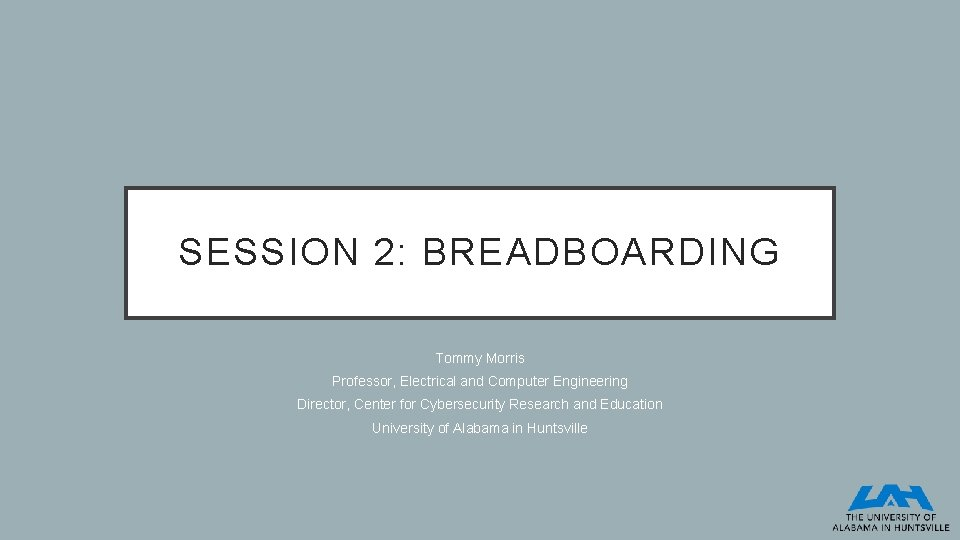SESSION 2: BREADBOARDING Tommy Morris Professor, Electrical and Computer Engineering Director, Center for Cybersecurity Research and Education University of Alabama in Huntsville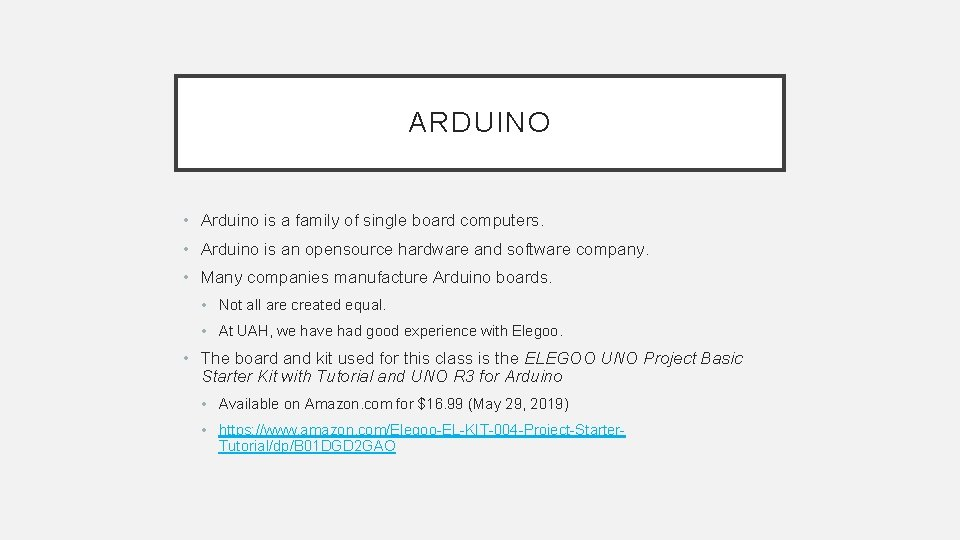ARDUINO • Arduino is a family of single board computers. • Arduino is an opensource hardware and software company. • Many companies manufacture Arduino boards. • Not all are created equal. • At UAH, we have had good experience with Elegoo. • The board and kit used for this class is the ELEGOO UNO Project Basic Starter Kit with Tutorial and UNO R 3 for Arduino • Available on Amazon. com for \$16. 99 (May 29, 2019) • https: //www. amazon. com/Elegoo-EL-KIT-004 -Project-Starter. Tutorial/dp/B 01 DGD 2 GAO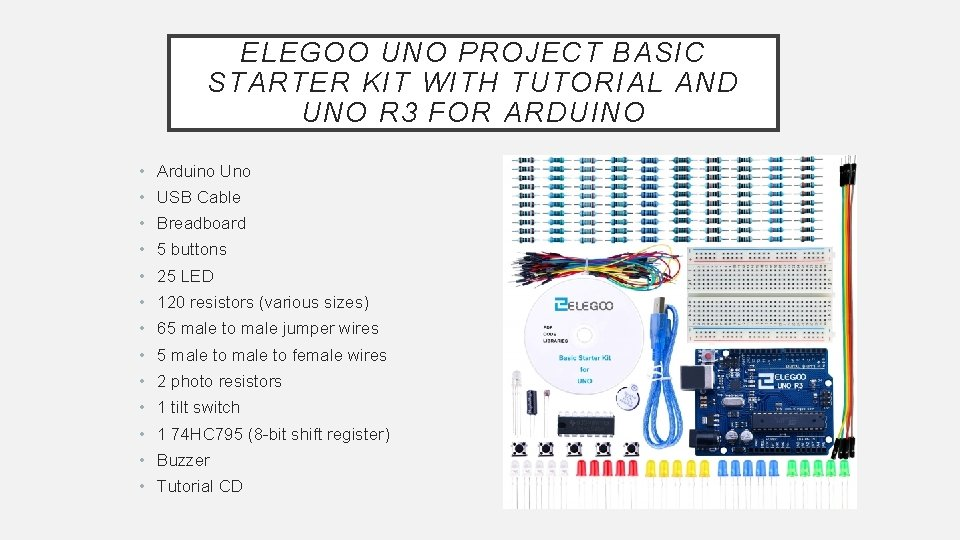ELEGOO UNO PROJECT BASIC STARTER KIT WITH TUTORIAL AND UNO R 3 FOR ARDUINO • Arduino Uno • USB Cable • Breadboard • 5 buttons • 25 LED • 120 resistors (various sizes) • 65 male to male jumper wires • 5 male to female wires • 2 photo resistors • 1 tilt switch • 1 74 HC 795 (8 -bit shift register) • Buzzer • Tutorial CDBREADBOARD CONNECTIVITY • Rows are labeled 1, 2, 3, …, 30 • Columns - labeled a, b, c, …, j • All pinholes on the same row in columns a-e are short circuited to one another • All pinholes on the same row in columns f-j are short circuited to one another • + and - rails are available on the left and right sides of the board. • For each side (left and right) all rows of the + column are short circuited to one another • For each side (left and right) all rows of the column are short circuited to one another • The left and right sides are not connected to each other short circuit examples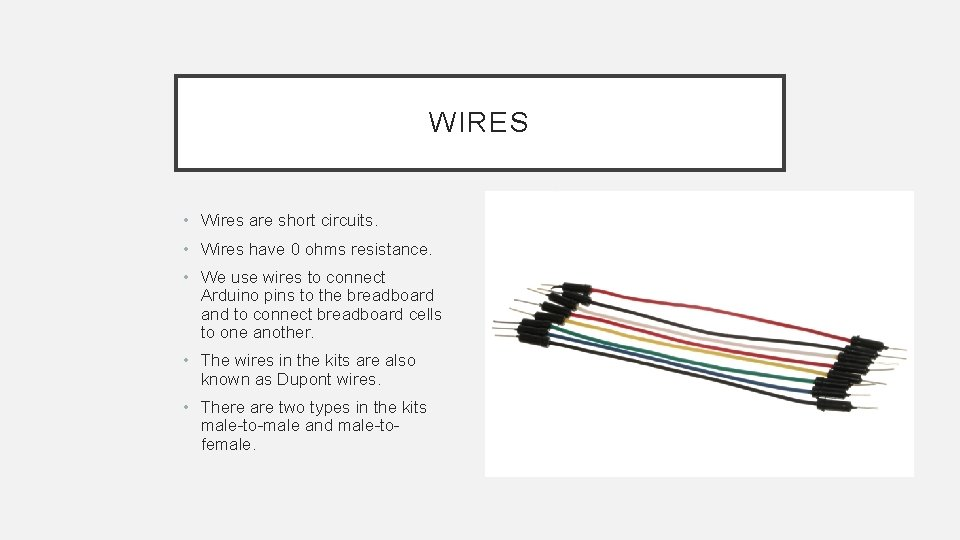WIRES • Wires are short circuits. • Wires have 0 ohms resistance. • We use wires to connect Arduino pins to the breadboard and to connect breadboard cells to one another. • The wires in the kits are also known as Dupont wires. • There are two types in the kits male-to-male and male-tofemale.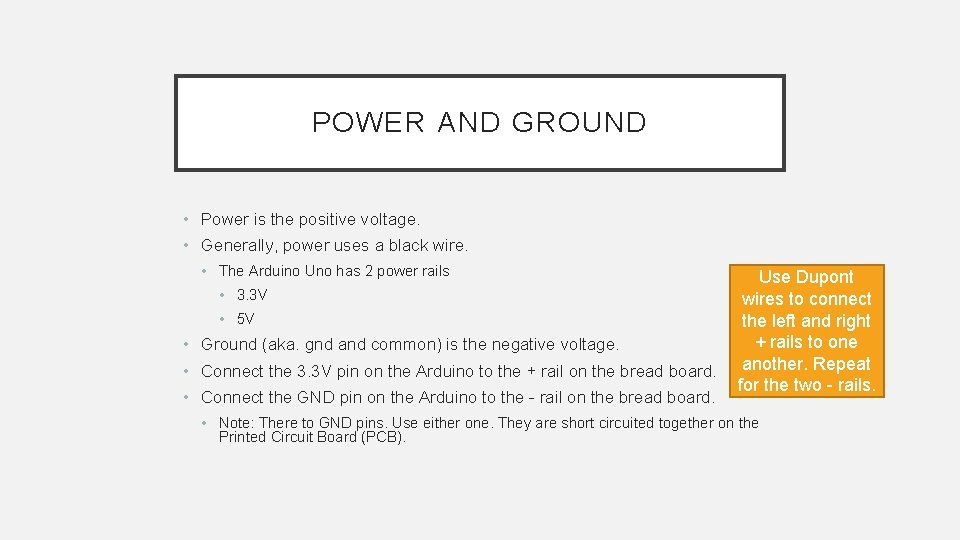POWER AND GROUND • Power is the positive voltage. • Generally, power uses a black wire. • The Arduino Uno has 2 power rails • 3. 3 V • 5 V • Ground (aka. gnd and common) is the negative voltage. • Connect the 3. 3 V pin on the Arduino to the + rail on the bread board. • Connect the GND pin on the Arduino to the - rail on the bread board. Use Dupont wires to connect the left and right + rails to one another. Repeat for the two - rails. • Note: There to GND pins. Use either one. They are short circuited together on the Printed Circuit Board (PCB).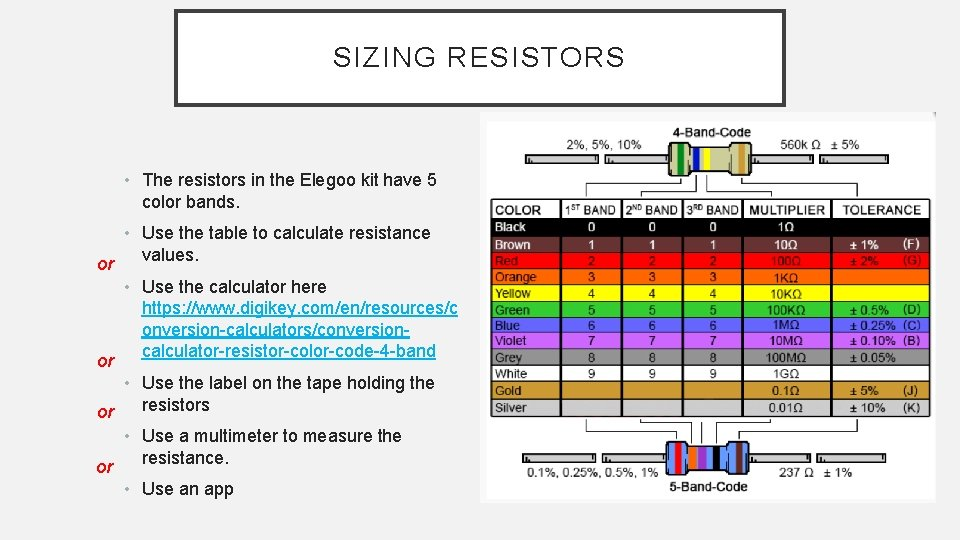SIZING RESISTORS • The resistors in the Elegoo kit have 5 color bands. or or • Use the table to calculate resistance values. • Use the calculator here https: //www. digikey. com/en/resources/c onversion-calculators/conversioncalculator-resistor-color-code-4 -band • Use the label on the tape holding the resistors or • Use a multimeter to measure the resistance. or • Use an appWHAT IS A RESISTOR? • Resistors limit current. They limit the number of electrons that can Arduino has 2 pass through a medium. voltages: 3. 3 V • Resistors are measured in units of Ohms. and 5 V. These don’t • Ohms law states: change. • V = RI : Voltage (V) = Resistance (R) * Current (I) • For a given (constant) voltage, as resistance increases current decreases. • Resistance is also known as impedance. • For DC (Direct Current) circuits resistance and impedance are the same. • Resistance is abbreviated R and impedance Z.RESISTOR SYMBOLS • Sometimes resistors are labeled R 1, R 2, . . . (or Z 1, Z 2, …) • Sometimes resistors are labeled with values.BUTTONS • Push button switches • Buttons are normally open circuits. • This means there is infinite resistance across the terminals when open (not pressed) • The terminals are electrically isolated from one another • When pressed the circuit is closed • This means there is 0 resistance across the terminals • The terminals are electrically short circuited to another when closed (pressed)BUTTONS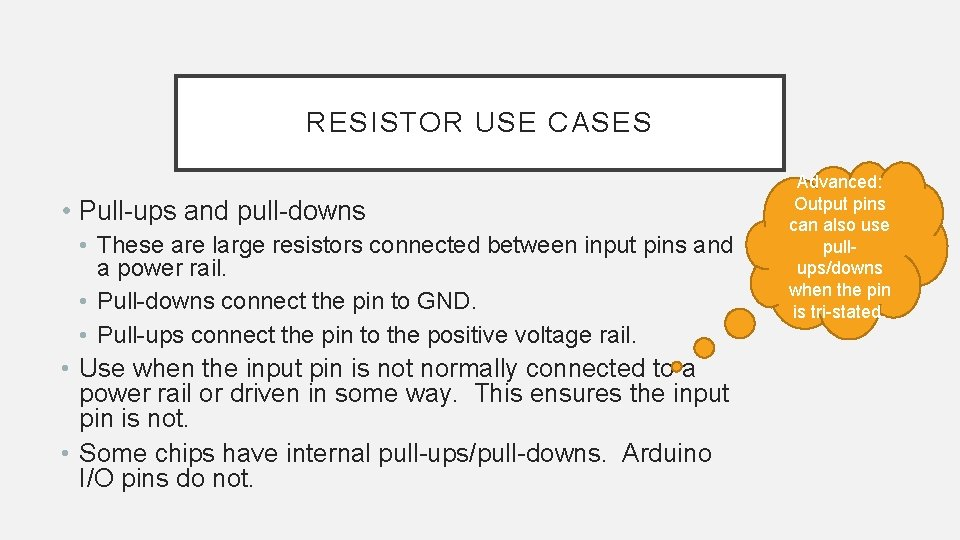RESISTOR USE CASES • Pull-ups and pull-downs • These are large resistors connected between input pins and a power rail. • Pull-downs connect the pin to GND. • Pull-ups connect the pin to the positive voltage rail. • Use when the input pin is not normally connected to a power rail or driven in some way. This ensures the input pin is not. • Some chips have internal pull-ups/pull-downs. Arduino I/O pins do not. Advanced: Output pins can also use pullups/downs when the pin is tri-stated.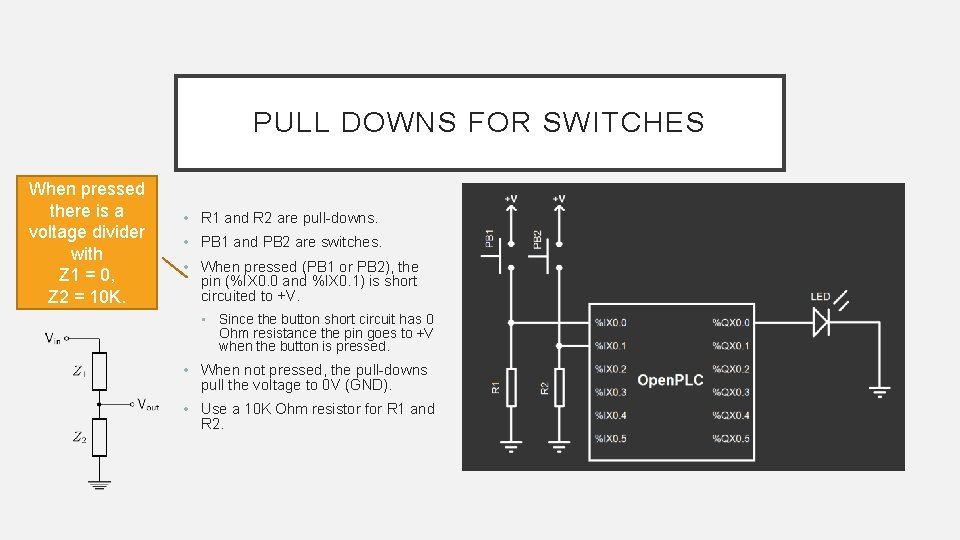PULL DOWNS FOR SWITCHES When pressed there is a voltage divider with Z 1 = 0, Z 2 = 10 K. • R 1 and R 2 are pull-downs. • PB 1 and PB 2 are switches. • When pressed (PB 1 or PB 2), the pin (%IX 0. 0 and %IX 0. 1) is short circuited to +V. • Since the button short circuit has 0 Ohm resistance the pin goes to +V when the button is pressed. • When not pressed, the pull-downs pull the voltage to 0 V (GND). • Use a 10 K Ohm resistor for R 1 and R 2.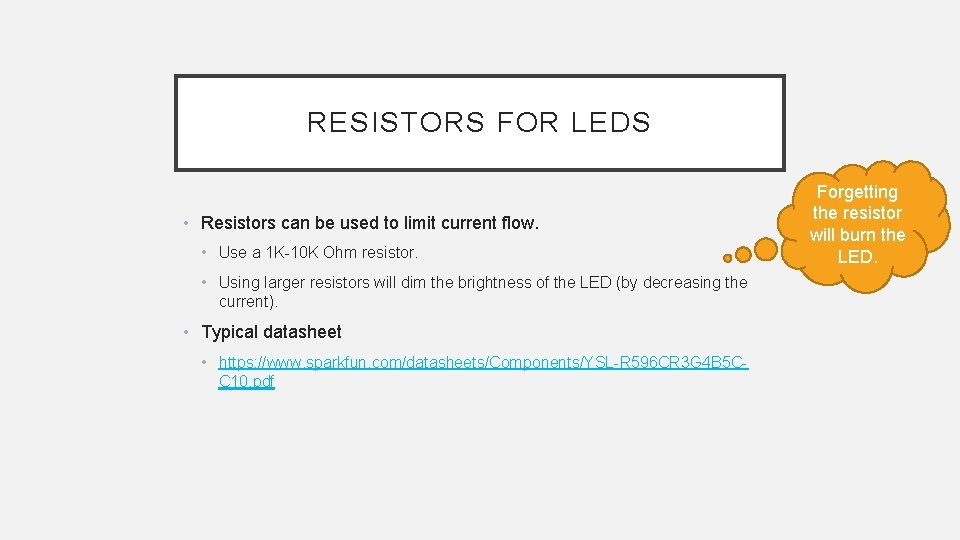RESISTORS FOR LEDS • Resistors can be used to limit current flow. • Use a 1 K-10 K Ohm resistor. • Using larger resistors will dim the brightness of the LED (by decreasing the current). • Typical datasheet • https: //www. sparkfun. com/datasheets/Components/YSL-R 596 CR 3 G 4 B 5 CC 10. pdf Forgetting the resistor will burn the LED.LED • Light Emitting Diode (LED)